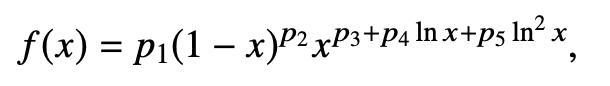# Fitting 5p function to pseudodata

Hello,
I am trying to fit a five parameter function to pseudo data, but for some reason I get the warning
`Warning in <ROOT::Math::ROOT::Math::GausIntegrator>: Failed to reach the desired tolerance ; maxtol = 1e-09`
I think it has to do with the function I am using. I attached the function and the file._ROOT Version: 6.23/01

Your function is singular at x=0 and invalid at x>1. Define it in the interval say 1.e-9<=x<=1

Use

``````TF1 *parabola_5 = new TF1("para_five","*((1-x)**)*x**(+*TMath::Log(x)+*(TMath::Log(x))^2)",1.e-9,1);
parabola_5->SetParameters(a,b,c,d,f);
parabola_5->SetParNames("a","b","c","d","f");
``````

and

``````for (int i=1; i<=5000;++i) histo.Fill(parabola_5->GetRandom(1.e-9,1));
``````